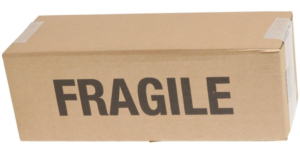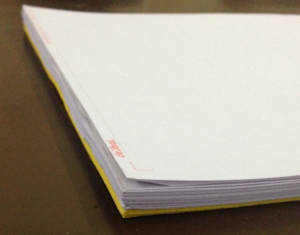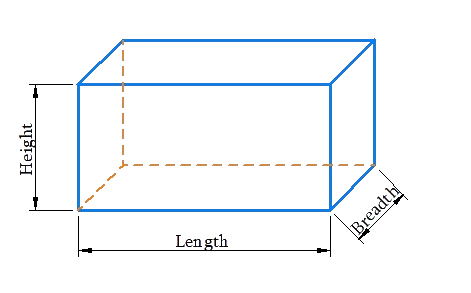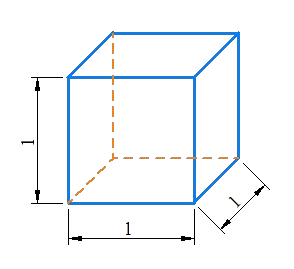# Cuboid Basics

Go back to  'Mensuration-Solids'

We have all seen examples of cuboids in daily life. Matchboxes and cardboard boxes are examples of cuboids:A cuboid is a three-dimensional analogue of a rectangle in two dimensions. For example, an A4 sheet of paper is a two-dimensional rectangle (approximately, if you ignore the finite thickness it has), but when you stack a lot of A4 sheets together, you have a three-dimensional cuboid:Clearly, a cuboid is characterized by three parameters of length, which are generally named: length, breadth and height:Note that there is no strict rule according to which an edge of a cuboid should be named its length, breadth or height. However, generally speaking, it is understood that the height represents the length of any vertical edge, if the cuboid is placed flat on a table, and then the length is taken to be the larger of the two dimensions of a horizontal face of the cuboid.

The dimensions of a cuboid will generally be denoted by l for length, b for breadth and h for height. Suppose that a cuboid has the dimensions 5 cm, 4 cm and 2 cm. We will say that it is a 5 cm × 4 cm × 2 cm cuboid.

Visualize a cuboid in your mind, with dimensions l, b and h. Observe that this cuboid will have: 6 faces, 12 edges and 8 vertices. The dimensions of the faces of the cuboid will be as follows:

• Two (opposite) faces will have the dimensions l and b.

• Two (opposite) faces have the dimensions b and h.

• The third pair of (opposite) faces will have the dimensions l and h.

A cube is a cuboid for which all the three dimensions are equal. The figure below shows a cube of side l units (each dimension is l units):Mensuration and Solids
Mensuration and Solids
grade 10 | Questions Set 2
Mensuration and Solids
Mensuration and Solids
grade 10 | Questions Set 1